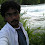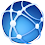## Goal:

How to understand PageRank algorithm in scala on Spark.

Spark 1.5.2
scala 2.10.4

## Algorithm:

The PageRank algorithm outputs a probability distribution used to represent the likelihood that a person randomly clicking on links will arrive at any particular page.
1. Initialize each page’s rank to 1.0.
2. On each iteration, have page p send a contribution of rank(p)/numNeighbors(p) to its neighbors (the pages it has links to).
3. Set each page’s rank to 0.15 + 0.85 * contributionsReceived.
The last two steps repeat for several iterations, during which the algorithm will con‐ verge to the correct PageRank value for each page. In practice, it’s typical to run about 10 iterations.

## Sample Data:

Above diagram shows there are 4 web pages with theirs outbound&inbound links.
If we consider the outbound links as a data type (String, List(String)), then data should be:
```("MapR",List("Baidu","Blogger")),
("Baidu", List("MapR"),

## Solution:

### 1. Initialize each page’s rank to 1.0.

```val links = sc.parallelize(List(("MapR",List("Baidu","Blogger")),("Baidu", List("MapR")),("Blogger",List("Google","Baidu")),("Google", List("MapR")))).partitionBy(new HashPartitioner(4)).persist()
var ranks = links.mapValues(v => 1.0)```

### 2. On each iteration, have page p send a contribution of rank(p)/numNeighbors(p) to its neighbors (the pages it has links to).

`val contributions = links.join(ranks).flatMap { case (url, (links, rank)) => links.map(dest => (dest, rank / links.size)) }`
See above contributions in red, and it matches the calculations using scala:
```scala> contributions.collect
res26: Array[(String, Double)] = Array((MapR,1.0), (Baidu,0.5), (Blogger,0.5), (Google,0.5), (Baidu,0.5), (MapR,1.0))```

### 3. Set each page’s rank to 0.15 + 0.85 * contributionsReceived.

`val ranks = contributions.reduceByKey((x, y) => x + y).mapValues(v => 0.15 + 0.85*v) `
After 1st iteration, current pagerank value for each page is:
```scala> ranks.collect
res27: Array[(String, Double)] = Array((Google,0.575), (MapR,1.8499999999999999), (Blogger,0.575), (Baidu,1.0))```

Then the last two steps repeat for several iterations.

Note: For the basic scala transformation syntax and examples, please refer to this scala cheat sheet.

1.Thanks very much.

2.Thanks for nice example

3.Thanks for nice explanation
how to replace the list with values assigned in other rdd
our list is(Google,List(MapR)),(MapR,List(Baidu,Blogger)....instead of this i want their values to be attached

1.I do not quite understand the ask. Could you share:
1. What is the input RDD
2. What is the output RDD you want

2.instead of value set 1.0 to every vertex set to number of neighbor links like(Google has 1.0) and (MapR has 0.5 (2 neighbors))
(Blogger also 0.5) (Baidu is 1.0) I got this from your code
I want the rdd like this
vertexname,ownprobability,neighbors probability since Google has only one neighbor and its probability is also 1.0
MapR has probability 1.0 and its neighbors probability are 0.5 and 0.5

4.Why does it required? - reduceByKey((x, y) => x + y)
Page ids are unique. Sorry I am new to scala and trying to learn.

1.That is to add the the values of the same key together.
For example, (MapR,1.0) + (MapR,1.0) ===> (MapR,2.0)

5.Small effort to pride the complete code:

import org.apache.spark.HashPartitioner